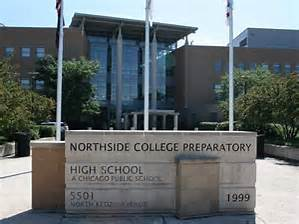# Northside's Selective Enrollment Open House

## Student and Parent Volunteers Needed

Saturday, October 20
11:00 am - 2:00 pm

Please encourage your students to sign up to help with the Open House. Student volunteers are needed from 9:00 am - 3:00 pm. There will be 2 training sessions: October 3 and 17. Students will be able to pick from X or Y sessions. On the day of the Open House, students will show families around, answer questions, and have the opportunity to share what they love about Northside. Pizza and snacks will be provided and students will earn service learning hours. Please encourage students to fill out this FORM to sign up.

We also need 100 adult volunteers to work this busy but super-fun event! Parents help answer questions and direct crowds in the gym, outside and all over the building. You will need to be registered as a Level II volunteer if you have not done so already. It very easy to do. Please see details below under CPS Volunteer Requirements.

 table div table+table+table+table div table{width:100%;padding:0}table div table+table+table+table div table img{width:96.23%;padding:0;float:none}table div table+table+table+table div table td{width:100%;padding:0 1.88% 18px}/* styles */# Parking Lot Safety

Parents, please remember that ALL VEHICLES MUST EXIT ONTO KEDZIE. You may enter the parking lot from Kedzie or Bryn Mawr, but regardless of where you enter, all cars must exit onto Kedzie. For the safety of our students and the rest of the Northside community, please do not exit onto Bryn Mawr.

 table div table+table+table+table+table+table div table{width:100%;padding:0}table div table+table+table+table+table+table div table img{width:96.23%;padding:0;float:none}table div table+table+table+table+table+table div table td{width:100%;padding:0 1.88% 18px}/* styles */# Annual Appeal Mailing

Thursday, October 4
4:15 - 6:15 pm
Faculty Lounge

We're kicking off our Annual Appeal and need folks to label and stuff envelopes for the mailing that goes to all NCP families and friends. No skills required and guaranteed to be a good time! To sign up, click HERE.

 table div table+table+table+table+table+table+table+table+table div table{width:100%;padding:0}table div table+table+table+table+table+table+table+table+table div table img{width:96.23%;padding:0;float:none}table div table+table+table+table+table+table+table+table+table div table td{width:100%;padding:0 1.88% 18px}/* styles */# Volunteers Still Needed

Make a commitment to Northside by offering your time to work with other volunteers in one of these rewarding, on-going positions. Email Friends of Northside to indicate your interest or ask questions.

## Eblast Co-Editor

Compose and distribute This Week at Northside and prepare other standalone blasts as requested.

## Graphic Designer

Provide professional guidance and materials for various design needs throughout the year.

## Website Editor

Keep the Friends of Northside website current and enhance its visual appeal.

## Database Manager

Maintain the Friends of Northside CRM (customer relationship management) database and develop meaningful reports.

## Teachers' Lounge Volunteer

Help stock and maintain the Teachers’ Lounge. This is a great low-commitment way to give back to NCP, only 30-45 minutes once a week, you pick the day and time. Call or text Eileen at 617-642-6267 for details or donate goods HERE.

## Join the Gala Committee

The Gala is NCP’s major spring fundraiser. Get involved, get to know NCP staff and parents and make a profound impact on Northside College Prep.

When is the Gala?
Friday, March 8, 2019

What’s the time commitment for the team?

What roles are available?
* Entertainment Chair - one or two people to determine and arrange the entertainment for the Gala.
* Auction Item Solicitors - at least five people to solicit auction items. It requires just a few hours a week and can be done in person or via phone or email.

 table div table+table+table+table+table+table+table+table+table+table+table+table div table{width:100%;padding:0}table div table+table+table+table+table+table+table+table+table+table+table+table div table img{width:96.23%;padding:0;float:none}table div table+table+table+table+table+table+table+table+table+table+table+table div table td{width:100%;padding:0 1.88% 18px}/* styles */# CPS Volunteer Requirements

According to CPS policy, anybody who is going to be in contact with students must be approved as a CPS volunteer. There are two levels of volunteers, Level 1 and Level 2.

## Level II Volunteers

(This process can be completed in a day & is required even of volunteers for Friends of Northside events)
A fingerprint criminal background check is not required for Level II Volunteers.

A Level II Volunteer is:

1. A parent providing supervised volunteer service in their child’s school or classroom for less than 10 hours/week;
2. Any individual providing volunteer service for less than 5 hours/week at a school where s/he does not have a child enrolled;
3. An individual providing incidental volunteer service with no ongoing individualized interaction with a student(s) including those who speak at a class/assembly, judge academic competitions, give musical performance, participate in the “Principal for a Day” program, job shadowing event or other one-time event provided where there is direct supervision of the activity/event by regular school employees;
4. A parent accompanying his/her child’s class on a one-day field trip or another type of extracurricular activity that does not involve an overnight stay;
5. An individual providing volunteer service on projects/activities involving no or nominal contact with children (home-based volunteer activities, volunteers serving at Central or Network office).
 1 A parent providing supervised volunteer service in their child’s school or classroom for less than 10 hours/week;
 2 Any individual providing volunteer service for less than 5 hours/week at a school where s/he does not have a child enrolled;
 3 An individual providing incidental volunteer service with no ongoing individualized interaction with a student(s) including those who speak at a class/assembly, judge academic competitions, give musical performance, participate in the “Principal for a Day” program, job shadowing event or other one-time event provided where there is direct supervision of the activity/event by regular school employees;
 4 A parent accompanying his/her child’s class on a one-day field trip or another type of extracurricular activity that does not involve an overnight stay;
 5 An individual providing volunteer service on projects/activities involving no or nominal contact with children (home-based volunteer activities, volunteers serving at Central or Network office).

## Level I Volunteers

(Please allow 8-12 weeks for the entire process)
A fingerprint criminal background check is required for all Level I Volunteers.

A Level I volunteer is:

1. A parent providing volunteer service in their child’s school or classroom for 10 or more hours/week;
2. An individual providing volunteer service for 5 or more hours/week at a school where s/he does not have a child enrolled;
3. Any individual chaperoning an overnight school-sponsored trip, regardless of the hours/week that the volunteer serves (See also the Student Travel Policy).
 1 A parent providing volunteer service in their child’s school or classroom for 10 or more hours/week;
 2 An individual providing volunteer service for 5 or more hours/week at a school where s/he does not have a child enrolled;
 3 Any individual chaperoning an overnight school-sponsored trip, regardless of the hours/week that the volunteer serves (See also the Student Travel Policy).

To become a volunteer, please visit CPS' Volunteer website and follow the instructions. There is also a link on Northside's website on the Parents page Northside Volunteers.

Assistant Principal Kaitlin O'Hare is Northside's Volunteer Coordinator. After applying to become a volunteer, you must visit the school and show Ms. O'Hare your ID, so she can approve you to move forward in the volunteer process. For Level II volunteers, that is the only step you must take. For Level 1, you will receive further instructions from CPS.

 table div table+table+table+table+table+table+table+table+table+table+table+table+table+table div table{width:100%;padding:0}table div table+table+table+table+table+table+table+table+table+table+table+table+table+table div table img{width:96.23%;padding:0;float:none}table div table+table+table+table+table+table+table+table+table+table+table+table+table+table div table td{width:100%;padding:0 1.88% 18px}/* styles */# This Week in Sports

Come to a game or match and support our student athletes!

## Men's Softball

▪ Monday, October 1, 4:30 pm vs Payton at Payton (V)
 ▪ Monday, October 1, 4:30 pm vs Payton at Payton (V)

## Women's Volleyball

▪ Tuesday, October 2, 5:00 (V) and 6:00 (SO) pm vs Ag-science at Ag-science
▪ Thursday, October 4, 4:30 (SO) and 5:30 (V) pm vs Lake Forest Academy at NCP
▪ Saturday, October 6, time TBD, NCP Invite in the NCP Gym (SO)
▪ Saturday, October 6, 10:30 am vs Westinghouse at Amundsen HS (F)
▪ Saturday, October 6, 11:15 am vs Taft HS at Amundsen HS (F)
▪ Saturday, October 6, time TBD, CPS Playoffs at Lane Tech HS (F)
 ▪ Tuesday, October 2, 5:00 (V) and 6:00 (SO) pm vs Ag-science at Ag-science
 ▪ Thursday, October 4, 4:30 (SO) and 5:30 (V) pm vs Lake Forest Academy at NCP
 ▪ Saturday, October 6, time TBD, NCP Invite in the NCP Gym (SO)
 ▪ Saturday, October 6, 10:30 am vs Westinghouse at Amundsen HS (F)
 ▪ Saturday, October 6, 11:15 am vs Taft HS at Amundsen HS (F)
 ▪ Saturday, October 6, time TBD, CPS Playoffs at Lane Tech HS (F)

## Women's Swimming

▪ Tuesday, October 2, 5:00 pm vs UHigh at the University of Chicago
▪ Thursday, October 4, 5:00 pm vs Lane at NCP (V)
 ▪ Tuesday, October 2, 5:00 pm vs UHigh at the University of Chicago
 ▪ Thursday, October 4, 5:00 pm vs Lane at NCP (V)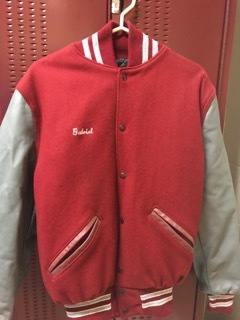# Order Your Northside Athletic Jacket Now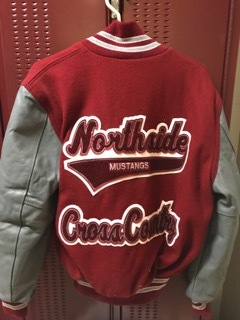Contact Mr. Pagani with any questions.

Deadline to order: Wednesday, October 10

 table div table+table+table+table+table+table+table+table+table+table+table+table+table+table+table+table+table+table+table div table{width:100%;padding:0}table div table+table+table+table+table+table+table+table+table+table+table+table+table+table+table+table+table+table+table div table img{width:96.23%;padding:0;float:none}table div table+table+table+table+table+table+table+table+table+table+table+table+table+table+table+table+table+table+table div table td{width:100%;padding:0 1.88% 18px}/* styles */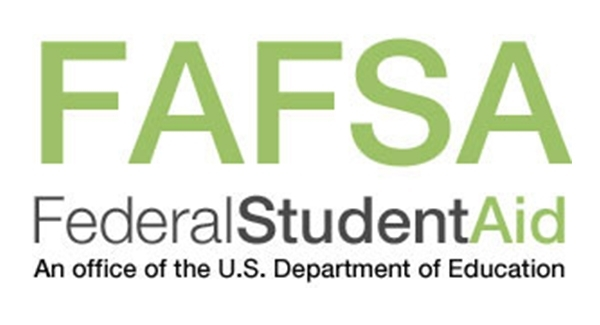# FAFSA Completion Workshop

Tuesday, October 16
6:00 - 8:00 pm
NCP Library, 2nd Floor

The FAFSA form should be filled out as soon as possible because it helps to determine your eligibility for federal, state, local and college scholarships, grants and loans. If you have not completed your FAFSA form or have questions, this workshop will provide students and parents support with their financial aid forms.

For students attending college in the 2019-2020 school year, you will need financial information from 2017.

· Your Social Security card. It is important that you enter your Social Security Number correctly!

· Your (and if married, your spouse's) 2017 federal income tax return and any other records so that you can prepare your FAFSA carefully and correctly

Contact Mr. Alexander Hughes in the counseling office if you have any questions. He can be reached at 773-534-3974 or ACHughes2@cps.edu.

 table div table+table+table+table+table+table+table+table+table+table+table+table+table+table+table+table+table+table+table+table+table div table{width:100%;padding:0}table div table+table+table+table+table+table+table+table+table+table+table+table+table+table+table+table+table+table+table+table+table div table img{width:96.23%;padding:0;float:none}table div table+table+table+table+table+table+table+table+table+table+table+table+table+table+table+table+table+table+table+table+table div table td{width:100%;padding:0 1.88% 18px}/* styles */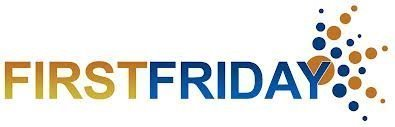/* styles */ Friday, October 5 8:00 - 10:00 am Panera Bread, 6059 N Lincoln Ave Friends of Northside gathers on the First Friday morning of every month for coffee and conversation. This casual get-together gives you a chance to meet and network with other parents. Feel free to come and go as it suits your schedule.
 table div table+table+table+table+table+table+table+table+table+table+table+table+table+table+table+table+table+table+table+table+table+table+table+table div table{width:100%;padding:0}table div table+table+table+table+table+table+table+table+table+table+table+table+table+table+table+table+table+table+table+table+table+table+table+table div table img{width:96.23%;padding:0;float:none}table div table+table+table+table+table+table+table+table+table+table+table+table+table+table+table+table+table+table+table+table+table+table+table+table div table td{width:100%;padding:0 1.88% 18px}/* styles */# No School

Columbus Day
Monday, October 8

 table div table+table+table+table+table+table+table+table+table+table+table+table+table+table+table+table+table+table+table+table+table+table+table+table+table+table div table{width:100%;padding:0}table div table+table+table+table+table+table+table+table+table+table+table+table+table+table+table+table+table+table+table+table+table+table+table+table+table+table div table img{width:96.23%;padding:0;float:none}table div table+table+table+table+table+table+table+table+table+table+table+table+table+table+table+table+table+table+table+table+table+table+table+table+table+table div table td{width:100%;padding:0 1.88% 18px}/* styles */# Other Important October Dates

Friday, October 5--First Friday
Monday, October 8--Columbus Day, no school
Thursday, October 11--Athletic Boosters Meeting
Tuesday, October 16--LSC Meeting
Tuesday, October 16--FAFSA Completion Workshop
Saturday, October 20--NCP Open House
Tuesday, October 23--FON Meeting
Thursday, October 25--Halloween Concert
Friday, October 26--Halloween Dance sponsored by the Latin Club

 table div table+table+table+table+table+table+table+table+table+table+table+table+table+table+table+table+table+table+table+table+table+table+table+table+table+table+table+table div table{width:100%;padding:0}table div table+table+table+table+table+table+table+table+table+table+table+table+table+table+table+table+table+table+table+table+table+table+table+table+table+table+table+table div table img{width:96.23%;padding:0;float:none}table div table+table+table+table+table+table+table+table+table+table+table+table+table+table+table+table+table+table+table+table+table+table+table+table+table+table+table+table div table td{width:100%;padding:0 1.88% 18px}/* styles */table.module-28{width:94.34%;padding:0}table div table+table+table+table+table+table+table+table+table+table+table+table+table+table+table+table+table+table+table+table+table+table+table+table+table+table+table+table+table div table{width:94.34%;float:none;margin-left:auto;margin-right:auto;padding:0}table div table+table+table+table+table+table+table+table+table+table+table+table+table+table+table+table+table+table+table+table+table+table+table+table+table+table+table+table+table div table a{border:0 none;text-decoration:none}table div table+table+table+table+table+table+table+table+table+table+table+table+table+table+table+table+table+table+table+table+table+table+table+table+table+table+table+table+table div table img{width:100%!important;border:0 none;text-decoration:none}table div table+table+table+table+table+table+table+table+table+table+table+table+table+table+table+table+table+table+table+table+table+table+table+table+table+table+table+table+table div table td{width:100%;padding:0}/* styles */
 /* styles */ Check out The HoofBeat, Northside's online newspaper, to get NCP news from the student perspective!
 table div table+table+table+table+table+table+table+table+table+table+table+table+table+table+table+table+table+table+table+table+table+table+table+table+table+table+table+table+table+table+table div table{width:100%;padding:0}table div table+table+table+table+table+table+table+table+table+table+table+table+table+table+table+table+table+table+table+table+table+table+table+table+table+table+table+table+table+table+table div table img{width:96.23%;padding:0;float:none}table div table+table+table+table+table+table+table+table+table+table+table+table+table+table+table+table+table+table+table+table+table+table+table+table+table+table+table+table+table+table+table div table td{width:100%;padding:0 1.88% 18px}/* styles */table.module-31{width:57.17%;padding:0}table div table+table+table+table+table+table+table+table+table+table+table+table+table+table+table+table+table+table+table+table+table+table+table+table+table+table+table+table+table+table+table+table div table{width:57.17%;float:none;margin-left:auto;margin-right:auto;padding:0}table div table+table+table+table+table+table+table+table+table+table+table+table+table+table+table+table+table+table+table+table+table+table+table+table+table+table+table+table+table+table+table+table div table a{border:0 none;text-decoration:none}table div table+table+table+table+table+table+table+table+table+table+table+table+table+table+table+table+table+table+table+table+table+table+table+table+table+table+table+table+table+table+table+table div table img{width:100%!important;border:0 none;text-decoration:none}table div table+table+table+table+table+table+table+table+table+table+table+table+table+table+table+table+table+table+table+table+table+table+table+table+table+table+table+table+table+table+table+table div table td{width:100%;padding:0}/* styles */

# What is FAN?

FAN is Family Action Network, an organization which connects parents, educators and professionals through collaborative programming that educates, inspires and positively impacts the broader community. Nearly every week during the school year there will be a dynamic and important speaker, discussing the issues that interest you.

FAN events are free to all.

# Comic Book Lessons That Shape How I Lead

Astro Teller, Ph.D.
Captain of Moonshots at X: The Moonshot Factory, the R&D lab of Alphabet, and Distignuished Alumni Award recipient, ETHS Class of 1988; Co-Founder, Cerebellum Capital

Monday, October 8, 7:00 pm
Evanston Township High School Auditorium
1600 Dodge Ave., Evanston

View flyer HERE

# Stamped from the Beginning

Ibram X. Kendi, Ph.D.
Professor of History and International Relations and Founding Director, Antiracist Research and Policy Center, American University; recipient of the 2016 National Book Award for Nonfiction for Stamped from the Beginning: The Definitive History of Racist Ideas in America

Friday, October 12, 7:00 pm
Evanston Township High School Auditorium
1600 Dodge Ave., Evanston

View flyer HERE

 table div table+table+table+table+table+table+table+table+table+table+table+table+table+table+table+table+table+table+table+table+table+table+table+table+table+table+table+table+table+table+table+table+table+table div table{width:100%;padding:0}table div table+table+table+table+table+table+table+table+table+table+table+table+table+table+table+table+table+table+table+table+table+table+table+table+table+table+table+table+table+table+table+table+table+table div table img{width:96.23%;padding:0;float:none}table div table+table+table+table+table+table+table+table+table+table+table+table+table+table+table+table+table+table+table+table+table+table+table+table+table+table+table+table+table+table+table+table+table+table div table td{width:100%;padding:0 1.88% 18px}/* styles */# FON Connect

Friends of Northside uses a customer/constituent relationship management (CRM) system known as FON Connect that allows our parent volunteer organizations to work from one database. It is not a CPS database and is completely different and separate from the CPS Parent Portal and Northside's EPay website.

FON Connect serves as the NCP Family Directory and allows users to review their donation history, update their contact information, register for upcoming events and more. Each parent has a separate account. Transactions, including donations, are recorded in the account of the parent who initiated the transaction or donation.

If you’re new to FON Connect, you'll need to set up a login and password. To do so:

 table div table+table+table+table+table+table+table+table+table+table+table+table+table+table+table+table+table+table+table+table+table+table+table+table+table+table+table+table+table+table+table+table+table+table+table+table div table{width:100%;padding:0}table div table+table+table+table+table+table+table+table+table+table+table+table+table+table+table+table+table+table+table+table+table+table+table+table+table+table+table+table+table+table+table+table+table+table+table+table div table img{width:96.23%;padding:0;float:none}table div table+table+table+table+table+table+table+table+table+table+table+table+table+table+table+table+table+table+table+table+table+table+table+table+table+table+table+table+table+table+table+table+table+table+table+table div table td{width:100%;padding:0 1.88% 18px}/* styles */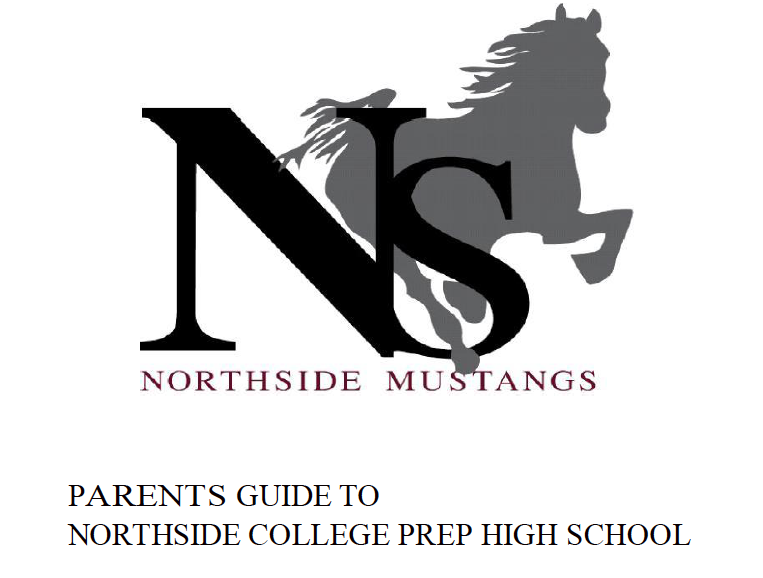# Navigating Northside

Navigating Northside, our parent handbook, is available on the school website under the Parents tab. Click HERE to access it directly.

 table div table+table+table+table+table+table+table+table+table+table+table+table+table+table+table+table+table+table+table+table+table+table+table+table+table+table+table+table+table+table+table+table+table+table+table+table+table+table div table{width:100%;padding:0}table div table+table+table+table+table+table+table+table+table+table+table+table+table+table+table+table+table+table+table+table+table+table+table+table+table+table+table+table+table+table+table+table+table+table+table+table+table+table div table img{width:96.23%;padding:0;float:none}table div table+table+table+table+table+table+table+table+table+table+table+table+table+table+table+table+table+table+table+table+table+table+table+table+table+table+table+table+table+table+table+table+table+table+table+table+table+table div table td{width:100%;padding:0 1.88% 18px}/* styles */# NCP Women's Varsity Cross Country Wins Medals at the Fleet Feet Invitational

Congratulations to Gwen Casey (junior), who placed 7th, Elia Ton-That (freshman) who placed 9th, Kendra Lachcik (senior) who placed 20th, and the entire women's cross country team for a great performance at the Fleet Feet Invitational hosted by Whitney Young HS at Washington Park on Saturday, September 22.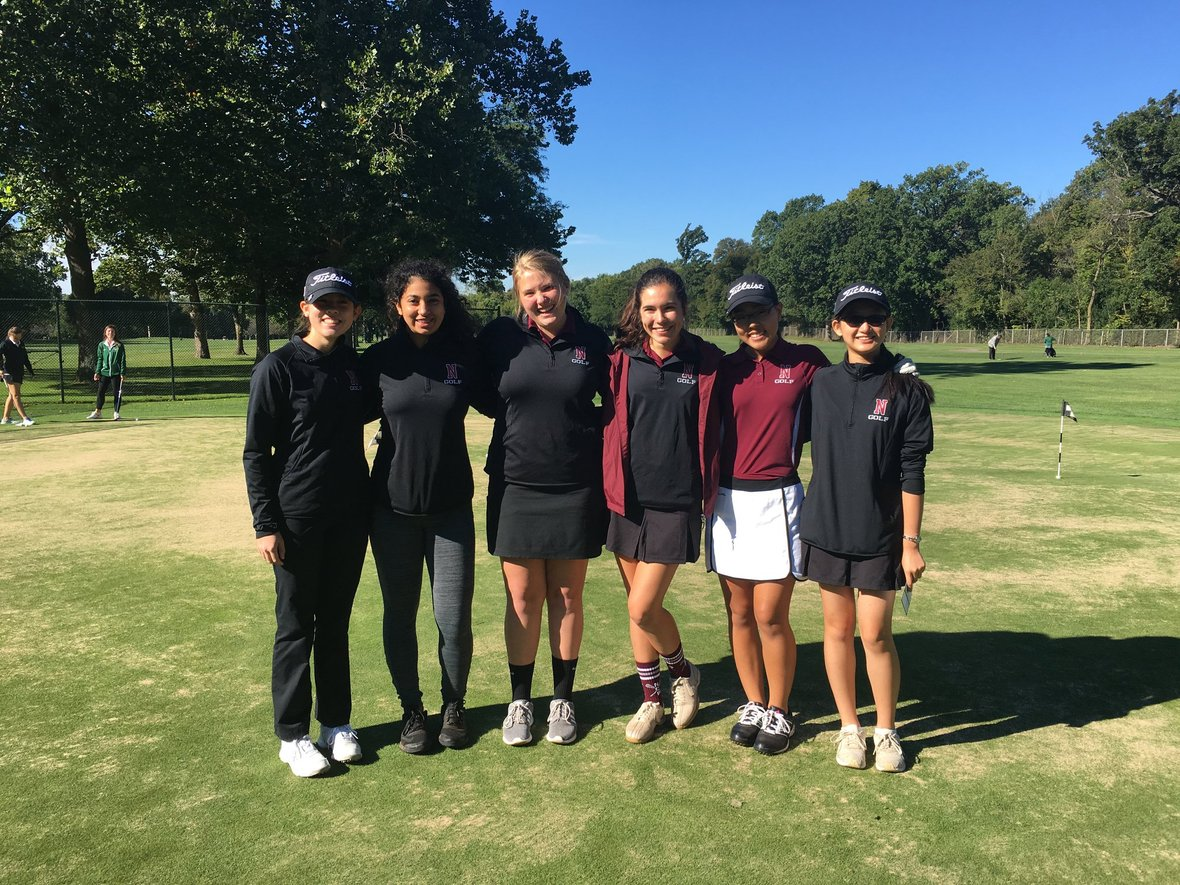# NCP Women's Golf Advances to CPS City Championships

Congratulations to the Women's Golf team who out scored Taft HS and tied Lane to secure a spot a the CPS City Championships. Both those schools have populations that are 3 and 4 times the size of NCP and a larger roster of players to choose from. NCP has a small but mighty team who achieve a lot despite their size.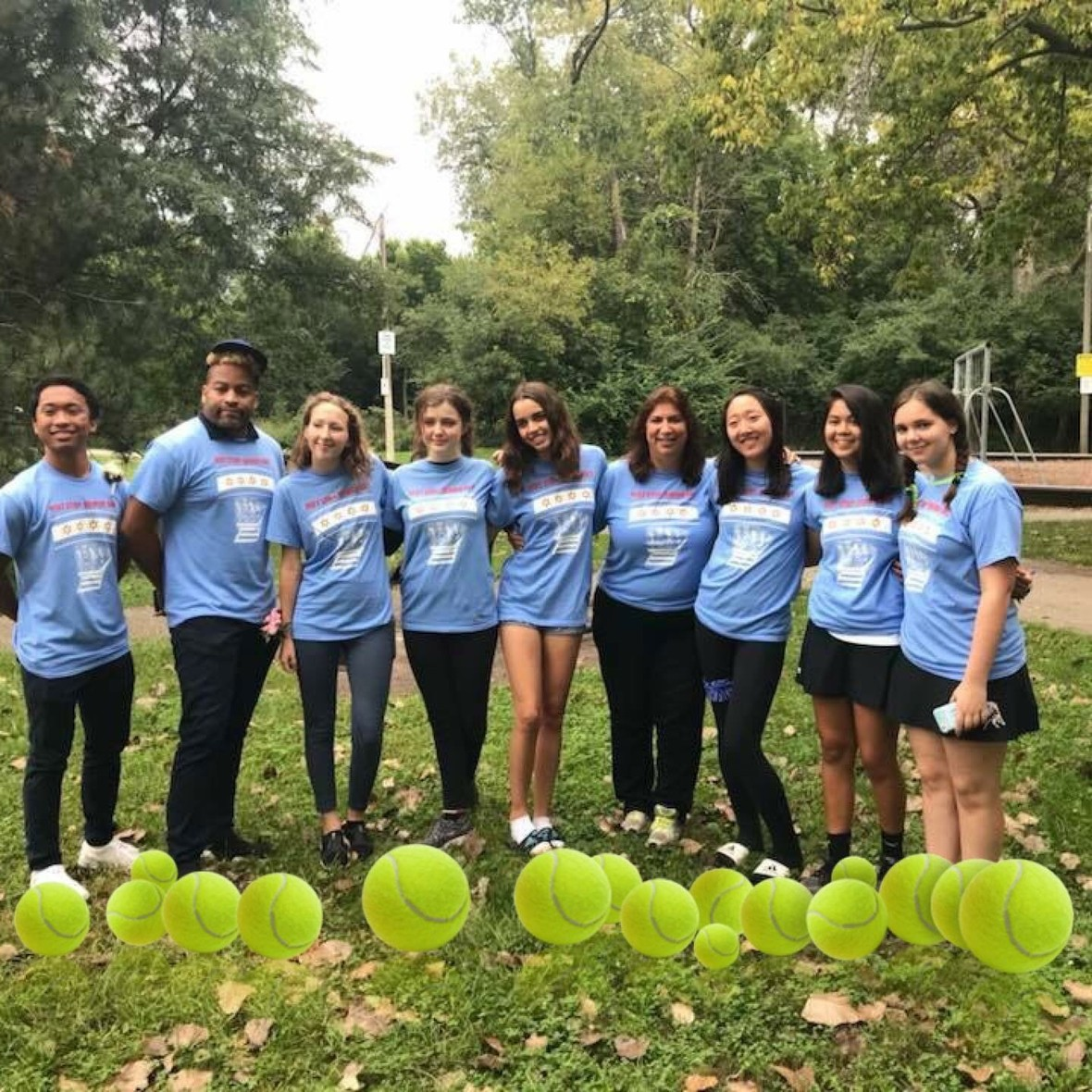# NCP Women's Tennis Qualifies for City Tournament

Congratulations to the women's tennis team for advancing to the city tournament. The team also celebrated Senior Day. Seven seniors, including 6 players and the team manager, are playing their last season at Northside. You will be missed!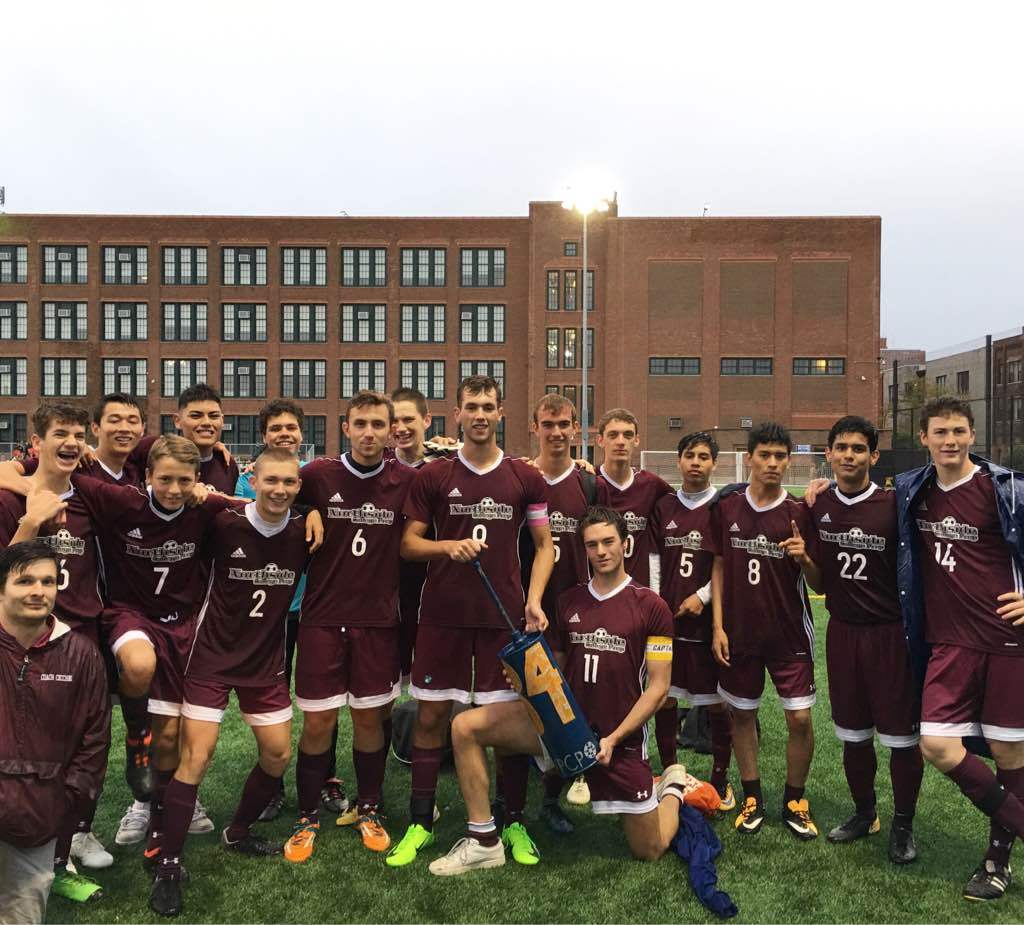# Men's Varsity Soccer Lands #1 Seed in Their State Regional; Beats Payton 5-0 in City Playoffs

Congratulations to the Northside men's soccer team for landing the number one seed in their regional for the state playoffs and beating rivals Walter Payton in the first round of the CPS tournament.

 table div table+table+table+table+table+table+table+table+table+table+table+table+table+table+table+table+table+table+table+table+table+table+table+table+table+table+table+table+table+table+table+table+table+table+table+table+table+table+table+table+table+table+table+table+table+table div table{width:100%;padding:0}table div table+table+table+table+table+table+table+table+table+table+table+table+table+table+table+table+table+table+table+table+table+table+table+table+table+table+table+table+table+table+table+table+table+table+table+table+table+table+table+table+table+table+table+table+table+table div table img{width:96.23%;padding:0;float:none}table div table+table+table+table+table+table+table+table+table+table+table+table+table+table+table+table+table+table+table+table+table+table+table+table+table+table+table+table+table+table+table+table+table+table+table+table+table+table+table+table+table+table+table+table+table+table div table td{width:100%;padding:0 1.88% 18px}/* styles */# Yale Information Sessions

An Associate Director of Undergraduate Admissions will discuss academic programs, residential colleges and campus life, admissions, and financial aid. Free to attend and all are welcome. Registration is recommended but not required.

Thursday, October 4, 06:30 - 08:00 pm
Niles West High School
5701 Oakton St, Skokie
Register HERE

Tuesday, October 9, 06:30 - 08:00 pm
Whitney M. Young Magnet High School
211 S Laflin St, Chicago
Register HERE

# 100 Black Men of Chicago, Inc.'s - 16th Annual College Scholarship Fair

Saturday, October 13, 9:00 am - 2:00 pm
University of Illinois, at Chicago - Pavilion
525 S Racine Ave

Since 2003, the 100BMC has hosted an annual College Scholarship Fair with up to 200 Colleges and Universities interacting with nearly 5000 students, parents and educational partners.

Students can register HERE.

# Tufts University Admissions: Who Gets In and Why

Sunday, October 14, 02:00 - 04:00 pm
DoubleTree by Hilton Hotel Chicago - North Shore Conference Center
9599 Skokie Blvd, Skokie

The program will highlight the following:
1. Academics and campus life at Tufts University
2. The different components of a college application and how those materials are evaluated by selective admissions offices
3. Tips on successful essay writing and the impact of an application supplement
4. The role of recommendations and interviews in the process
5. A case study designed to resemble the admissions process, featuring five real applications from prior Tufts applicants

Students can register HERE for the workshop.

# Upcoming College Visits at Northside

Monday, October 1
Butler University, 9:48 am
Mercer University, 9:48 am
University of Aberdeen, 10:38 am
University of Massachusetts-Lowell, 12:26 pm
North Park University, 3:15 pm
Washington & Jefferson College, 3:15 pm

Tuesday, October 2
University of Illinois at Springfield, 10:15 am
University of Maryland-College Park, 10:15 am
University of Glasgow, 10:38 am
Dominican University, 10:38 am
College of Mount Saint Vincent, 12:26 pm
Illinois Institute of Technology, 3:15 pm

Wednesday, October 3
Carnegie Mellon University, 10:30 am
Milwaukee School of Engineering, 11:22 am
Oberlin College, 11:22 am
University of California-Los Angeles, 3:15 pm

Thursday, October 4
University of California, San Diego, 9:48 am
Vanderbilt University, 11:36 am
University of Exeter, 11:36 am
Colgate University, 12:26 pm
Bentley University, 3:15 pm

Friday, October 5
University of Houston, 9:48 am
University of Manchester, 10:38 am
Hamilton College, 10:38 am

The list of college visits is updated frequently. For the most current information, parents of juniors and seniors should log into their parent account for Naviance.

 table div table+table+table+table+table+table+table+table+table+table+table+table+table+table+table+table+table+table+table+table+table+table+table+table+table+table+table+table+table+table+table+table+table+table+table+table+table+table+table+table+table+table+table+table+table+table+table+table+table+table+table+table div table{width:100%;padding:0}table div table+table+table+table+table+table+table+table+table+table+table+table+table+table+table+table+table+table+table+table+table+table+table+table+table+table+table+table+table+table+table+table+table+table+table+table+table+table+table+table+table+table+table+table+table+table+table+table+table+table+table+table div table img{width:96.23%;padding:0;float:none}table div table+table+table+table+table+table+table+table+table+table+table+table+table+table+table+table+table+table+table+table+table+table+table+table+table+table+table+table+table+table+table+table+table+table+table+table+table+table+table+table+table+table+table+table+table+table+table+table+table+table+table+table div table td{width:100%;padding:0 1.88% 18px}/* styles */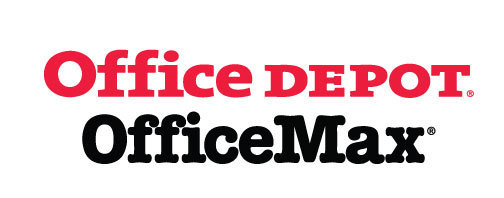# Give Back to Northside

Purchase school and office supplies at Office Depot and OfficeMax, and Northside can receive 5% back in credits or FREE supplies. Simply provide our school ID 70031171 at checkout, online or in stores. There’s no cost to you!

 table div table+table+table+table+table+table+table+table+table+table+table+table+table+table+table+table+table+table+table+table+table+table+table+table+table+table+table+table+table+table+table+table+table+table+table+table+table+table+table+table+table+table+table+table+table+table+table+table+table+table+table+table+table+table+table div table{width:100%;padding:0}table div table+table+table+table+table+table+table+table+table+table+table+table+table+table+table+table+table+table+table+table+table+table+table+table+table+table+table+table+table+table+table+table+table+table+table+table+table+table+table+table+table+table+table+table+table+table+table+table+table+table+table+table+table+table+table div table img{width:96.23%;padding:0;float:none}table div table+table+table+table+table+table+table+table+table+table+table+table+table+table+table+table+table+table+table+table+table+table+table+table+table+table+table+table+table+table+table+table+table+table+table+table+table+table+table+table+table+table+table+table+table+table+table+table+table+table+table+table+table+table+table div table td{width:100%;padding:0 1.88% 18px}/* styles */table.module-55{width:66.42%;padding:0}table div table+table+table+table+table+table+table+table+table+table+table+table+table+table+table+table+table+table+table+table+table+table+table+table+table+table+table+table+table+table+table+table+table+table+table+table+table+table+table+table+table+table+table+table+table+table+table+table+table+table+table+table+table+table+table+table div table{width:66.42%;float:none;margin-left:auto;margin-right:auto;padding:0}table div table+table+table+table+table+table+table+table+table+table+table+table+table+table+table+table+table+table+table+table+table+table+table+table+table+table+table+table+table+table+table+table+table+table+table+table+table+table+table+table+table+table+table+table+table+table+table+table+table+table+table+table+table+table+table+table div table a{border:0 none;text-decoration:none}table div table+table+table+table+table+table+table+table+table+table+table+table+table+table+table+table+table+table+table+table+table+table+table+table+table+table+table+table+table+table+table+table+table+table+table+table+table+table+table+table+table+table+table+table+table+table+table+table+table+table+table+table+table+table+table+table div table img{width:100%!important;border:0 none;text-decoration:none}table div table+table+table+table+table+table+table+table+table+table+table+table+table+table+table+table+table+table+table+table+table+table+table+table+table+table+table+table+table+table+table+table+table+table+table+table+table+table+table+table+table+table+table+table+table+table+table+table+table+table+table+table+table+table+table+table div table td{width:100%;padding:0}/* styles */

# Support Northside - Shop AmazonSmile

AmazonSmile is a simple and automatic way to support Northside every time you shop.

Please note that our AmazonSmile name is Friends of "North" "Side" (North Side - two words at AmazonSmile).

 table div table+table+table+table+table+table+table+table+table+table+table+table+table+table+table+table+table+table+table+table+table+table+table+table+table+table+table+table+table+table+table+table+table+table+table+table+table+table+table+table+table+table+table+table+table+table+table+table+table+table+table+table+table+table+table+table+table+table div table{width:100%;padding:0}table div table+table+table+table+table+table+table+table+table+table+table+table+table+table+table+table+table+table+table+table+table+table+table+table+table+table+table+table+table+table+table+table+table+table+table+table+table+table+table+table+table+table+table+table+table+table+table+table+table+table+table+table+table+table+table+table+table+table div table img{width:96.23%;padding:0;float:none}table div table+table+table+table+table+table+table+table+table+table+table+table+table+table+table+table+table+table+table+table+table+table+table+table+table+table+table+table+table+table+table+table+table+table+table+table+table+table+table+table+table+table+table+table+table+table+table+table+table+table+table+table+table+table+table+table+table+table div table td{width:100%;padding:0 1.88% 18px}/* styles */# Follow Us on Social Media

▪ Northside Prep Parents Facebook Page, a closed group for parents, by parents, to share their knowledge of Northside
▪ Friends of Northside Facebook Page, for news everyone can view
 ▪ Northside Prep Parents Facebook Page, a closed group for parents, by parents, to share their knowledge of Northside
 ▪ Friends of Northside Facebook Page, for news everyone can view
 table div table+table+table+table+table+table+table+table+table+table+table+table+table+table+table+table+table+table+table+table+table+table+table+table+table+table+table+table+table+table+table+table+table+table+table+table+table+table+table+table+table+table+table+table+table+table+table+table+table+table+table+table+table+table+table+table+table+table+table+table div table{width:100%;padding:0}table div table+table+table+table+table+table+table+table+table+table+table+table+table+table+table+table+table+table+table+table+table+table+table+table+table+table+table+table+table+table+table+table+table+table+table+table+table+table+table+table+table+table+table+table+table+table+table+table+table+table+table+table+table+table+table+table+table+table+table+table div table img{width:96.23%;padding:0;float:none}table div table+table+table+table+table+table+table+table+table+table+table+table+table+table+table+table+table+table+table+table+table+table+table+table+table+table+table+table+table+table+table+table+table+table+table+table+table+table+table+table+table+table+table+table+table+table+table+table+table+table+table+table+table+table+table+table+table+table+table+table div table td{width:100%;padding:0 1.88% 18px}/* styles */Northside’s website can be found HERE.

Friends of Northside's website can be found HERE.

 table div table+table+table+table+table+table+table+table+table+table+table+table+table+table+table+table+table+table+table+table+table+table+table+table+table+table+table+table+table+table+table+table+table+table+table+table+table+table+table+table+table+table+table+table+table+table+table+table+table+table+table+table+table+table+table+table+table+table+table+table+table+table div table{width:100%;padding:0}table div table+table+table+table+table+table+table+table+table+table+table+table+table+table+table+table+table+table+table+table+table+table+table+table+table+table+table+table+table+table+table+table+table+table+table+table+table+table+table+table+table+table+table+table+table+table+table+table+table+table+table+table+table+table+table+table+table+table+table+table+table+table div table img{width:96.23%;padding:0;float:none}table div table+table+table+table+table+table+table+table+table+table+table+table+table+table+table+table+table+table+table+table+table+table+table+table+table+table+table+table+table+table+table+table+table+table+table+table+table+table+table+table+table+table+table+table+table+table+table+table+table+table+table+table+table+table+table+table+table+table+table+table+table+table div table td{width:100%;padding:0 1.88% 18px}/* styles */